# Homology with compact support

A partially exact homology theory (cf. Homology theory) which satisfies the following axiom of compact support: For each elementof the-dimensional group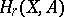of an arbitrary pair of spacesthere exists a compact pairsuch thatis contained in the image of the homomorphismwhich is induced by the inclusion. If the homology theoryis exact and has compact support, the following theorem is valid: For any element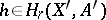which belongs to the kernel ofthere exists a compact pair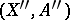such thatandbelongs to the kernel of the homomorphismAn exact theory has compact support if and only if for any pair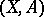the groupis the direct limit, where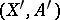runs through the compact pairs contained in. An exact homology theory with compact support is unique on the category of arbitrary (non-compact) polyhedral pairs for a given coefficient group and is equivalent to the singular theory. For a general homology theory, in addition to the groupthere is also the groupwhereare compact subpairs in. The singular homology group, having compact support, is isomorphic to. In spectral theory one also considers — in addition to the Aleksandrov–Čech homology groupsand the groups— the group which is the image of the natural homomorphism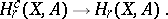This group, like the group, satisfies the axiom of compact support, but in spectral theory it is the latter group which has the name of homology with compact support. In spectral theory these three groups differ from one another, and each of them is the object of a duality theorem, both for a discrete and for a compact group of coefficients (cf. Duality in topology).